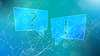# TechTopics No. 67

%dc component

With the changes in the circuit breaker rating structure introduced in 1999, the specification of interrupting capacity now includes a “%dc component.” What does this mean, and how does it relate to the asymmetrical interrupting factor, which is the “S-factor” from the previous standards?

In the 1979 and earlier editions of ANSI/IEEE C37.04 “Standard rating structure for AC high-voltage circuit breakers rated on a symmetrical current basis,” the asymmetrical interrupting capacity of the circuit breaker was given as a multiple of the symmetrical interrupting capacity, with the multiplier dependent on the contact part time of the circuit breaker. The multiplier was designated as the “S-factor” and the values were as shown in Table 1.

Table 1: S-factor
S-factor
Contact part time
Rated interrupting time
1.0
4 cycles
8 cycles
1.1
3 cycles
5 cycles
1.2
2 cycles
3 cycles
1.4
1 cycle
2 cycles

The value for contact part time includes the opening time of the circuit breaker, plus an allowance of 0.5 cycles for protective relay operating time. The relation of S-factor to contact part time is shown graphically in Figure 1.

Thus, the rms asymmetrical interrupting capacity of the circuit breaker was equal to: Iasymmetrical = (S-factor) x Isymmetrical.

Using an example circuit breaker rated 40 kA with a contact part time of 2.0 cycles, the asymmetrical interrupting capability would be 1.2 x 40 kA = 48 kA, under the rating structure of C37.04-1979.

In 1999, the new “constant kA” rating structure was introduced, and determining the asymmetrical interrupting capacity became a bit more difficult.

Table 2: Equivalent S-factor
S-factor (old)
Equivalent %dc component
Conduct part time
%dc component (new)
Equivalent S-factor
1.4
69.3%
1 cycle (16.67 ms)
69.1%
1.398
1.2
46.9%
2 cycles (33.33 ms)
47.8%
1.207
1.1
32.4%
3 cycles (50 ms)
33.0%
1.104
1.0
0%
4 cycles (66.67 ms)
22.8%
1.05

According to ANSI/IEEE C37.04-1999, clause 5.8.2.2, the asymmetrical interrupting capacity is calculated from the following equations:Iasymmetrical = Isymmetrical    √1+2 (%dc/100)2 %dc = 100e(-t/45) where t is the contact part time (in ms) of the circuit breaker (including ½-cycle relay time). The value of “45” in the denominator of the exponent is the circuit time constant of 45 ms, which is the time constant of decay of the dc component in a circuit with an X/R ratio of 17 on a 60 Hz system.

The latter equation is represented in Figure 2, using the time constant of 45 ms and X/R of 17.

From Figure 2 using a contact part of 33.3 ms (2 cycles on a 60 Hz basis), the %dc component would be 47.8 percent.

In turn, substituting this value in the equation for Iasymmetrical above, we obtain a value of 1.207 times Isymmetrical, which is essentially the value obtained with the older standards and the S-factor method.

If we compare the old S-factor to the modern %dc component and recognize that the S-factor scheme simplified the factors so they were “nice round numbers,” we see that the historic S-factor and the modern %dc component methods of specifying the asymmetrical interrupting capacity of a high-voltage circuit breaker are essentially equivalent.